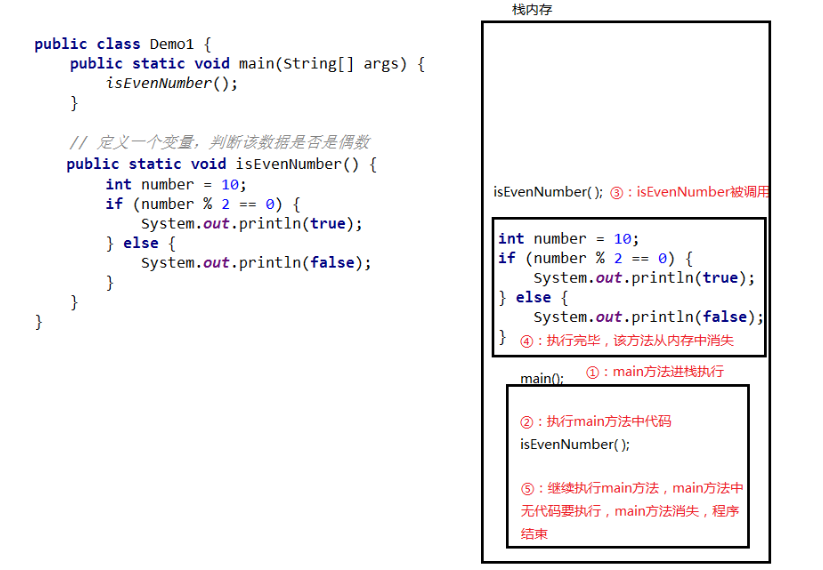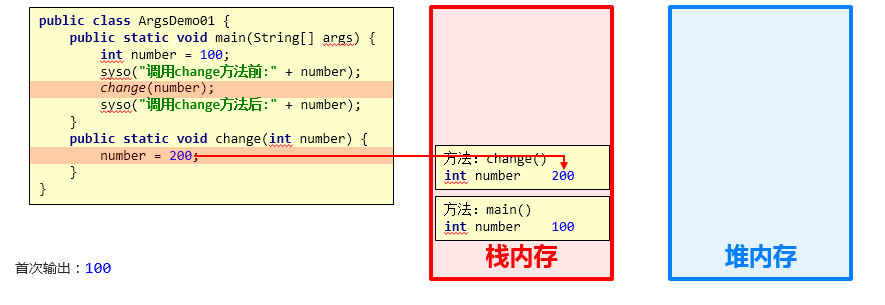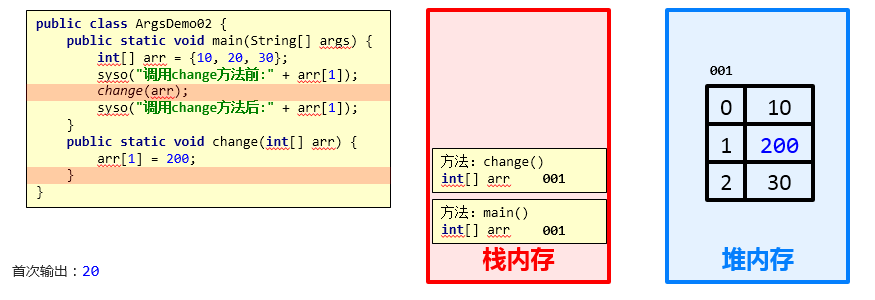## 1. 方法概述

### 1.1 方法的概念

​ 方法（method）是将具有独立功能的代码块组织成为一个整体，使其具有特殊功能的代码集

• 注意：
• 方法必须先创建才可以使用，该过程成为方法定义
• 方法创建后并不是直接可以运行的，需要手动使用后，才执行，该过程成为方法调用

## 2. 方法的定义和调用

### 2.1 无参数方法定义和调用

• 定义格式：

``````public static void 方法名 (   ) {

// 方法体;
}
``````
• 范例：

``````public static void method (    ) {

// 方法体;
}
``````
• 调用格式：

``````方法名();
``````
• 范例：

``````method();
``````
• 注意：

​ 方法必须先定义，后调用，否则程序将报错

### 2.2 方法调用过程图解• 总结：每个方法在被调用执行的时候，都会进入栈内存，并且拥有自己独立的内存空间，方法内部代码调用完毕之后，会从栈内存中弹栈消失。

### 2.3 无参数方法的练习

• 需求：设计一个方法用于打印两个数中的较大数
• 思路：
• ①定义一个方法，用于打印两个数字中的较大数，例如getMax()
• ②方法中定义两个变量，用于保存两个数字
• ③使用分支语句分两种情况对两个数字的大小关系进行处理
• ④在main()方法中调用定义好的方法
• 代码：
``````public class MethodTest {

public static void main(String[] args) {

//在main()方法中调用定义好的方法
getMax();
}

//定义一个方法，用于打印两个数字中的较大数，例如getMax()
public static void getMax() {

//方法中定义两个变量，用于保存两个数字
int a = 10;
int b = 20;

//使用分支语句分两种情况对两个数字的大小关系进行处理
if(a > b) {

System.out.println(a);
} else {

System.out.println(b);
}
}
}
``````

## 3. 带参数方法定义和调用

### 3.1 带参数方法定义和调用

• 定义格式：

参数：由数据类型和变量名组成 - 数据类型 变量名

参数范例：int a

``````public static void 方法名 (参数1) {

方法体;
}

public static void 方法名 (参数1, 参数2, 参数3...) {

方法体;
}
``````
• 范例：

``````public static void isEvenNumber(int number){

...
}
public static void getMax(int num1, int num2){

...
}
``````
• 注意：

``````方法定义时，参数中的数据类型与变量名都不能缺少，缺少任意一个程序将报错

方法定义时，多个参数之间使用逗号( ，)分隔
``````
• 调用格式：

``````方法名(参数)；

方法名(参数1,参数2);
``````
• 范例：

``````isEvenNumber(10);

getMax(10,20);
``````
• 方法调用时，参数的数量与类型必须与方法定义中的设置相匹配，否则程序将报错

### 3.2 形参和实参

1. 形参：方法定义中的参数

​ 等同于变量定义格式，例如：int number

1. 实参：方法调用中的参数

​ 等同于使用变量或常量，例如： 10 number

### 3.3 带参数方法练习

• 需求：设计一个方法用于打印两个数中的较大数，数据来自于方法参数 }
• 思路：
• ①定义一个方法，用于打印两个数字中的较大数，例如getMax()
• ②为方法定义两个参数，用于接收两个数字
• ③使用分支语句分两种情况对两个数字的大小关系进行处理
• ④在main()方法中调用定义好的方法（使用常量）
• ⑤在main()方法中调用定义好的方法（使用变量）
• 代码：
``````public class MethodTest {

public static void main(String[] args) {

//在main()方法中调用定义好的方法（使用常量）
getMax(10,20);
//调用方法的时候，人家要几个，你就给几个，人家要什么类型的，你就给什么类型的
//getMax(30);
//getMax(10.0,20.0);

//在main()方法中调用定义好的方法（使用变量）
int a = 10;
int b = 20;
getMax(a, b);
}

//定义一个方法，用于打印两个数字中的较大数，例如getMax()
//为方法定义两个参数，用于接收两个数字
public static void getMax(int a, int b) {

//使用分支语句分两种情况对两个数字的大小关系进行处理
if(a > b) {

System.out.println(a);
} else {

System.out.println(b);
}
}
}
``````

## 4. 带返回值方法的定义和调用

### 4.1 带返回值方法定义和调用

• 定义格式

``````public static 数据类型 方法名 ( 参数 ) {

return 数据 ;
}
``````
• 范例

``````public static boolean isEvenNumber( int number ) {

return true ;
}
public static int getMax( int a, int b ) {

return  100 ;
}
``````
• 注意：
• 方法定义时return后面的返回值与方法定义上的数据类型要匹配，否则程序将报错
• 调用格式

``````方法名 ( 参数 ) ;
数据类型 变量名 = 方法名 ( 参数 ) ;
``````
• 范例

``````isEvenNumber ( 5 ) ;
boolean  flag =  isEvenNumber ( 5 );
``````
• 注意：
• 方法的返回值通常会使用变量接收，否则该返回值将无意义

### 4.2 带返回值方法练习1

• 需求：设计一个方法可以获取两个数的较大值，数据来自于参数

• 思路：

• ①定义一个方法，用于获取两个数字中的较大数
• ②使用分支语句分两种情况对两个数字的大小关系进行处理
• ③根据题设分别设置两种情况下对应的返回结果
• ④在main()方法中调用定义好的方法并使用变量保存
• ⑤在main()方法中调用定义好的方法并直接打印结果
• 代码：

``````public class MethodTest {

public static void main(String[] args) {

//在main()方法中调用定义好的方法并使用变量保存
int result = getMax(10,20);
System.out.println(result);

//在main()方法中调用定义好的方法并直接打印结果
System.out.println(getMax(10,20));
}

//定义一个方法，用于获取两个数字中的较大数
public static int getMax(int a, int b) {

//使用分支语句分两种情况对两个数字的大小关系进行处理
//根据题设分别设置两种情况下对应的返回结果
if(a > b) {

return a;
} else {

return b;
}
}
}
``````

### 4.3 带返回值方法练习2

​ 定义一个方法，求一家商场每个季度的营业额。根据方法结果再计算出全年营业额。

``````package com.itheima.demo;

public class MethodDemo9 {

public static void main(String[] args) {

/*需求：定义一个方法，求一家商场每个季度的营业额。
根据方法结果再计算出全年营业额。*/
int sum1 = getSum(10, 20, 30);
int sum2 = getSum(10, 20, 30);
int sum3 = getSum(10, 20, 30);
int sum4 = getSum(10, 20, 30);

int sum = sum1 + sum2 + sum3 + sum4;
System.out.println(sum);

}

//心得：
//1.我要干嘛？  决定了方法体   每个季度的营业额
//2.我干这件事情，需要什么才能完成？ 决定了形参 需要三个月的营业额 a b c
//3.我干完这件事情，看调用处是否需要使用方法的结果。   决定了返回值
//如果需要使用，那么必须返回
//如果不需要使用，可以返回也可以不返回
public static int getSum(int month1,int month2,int month3){

int sum = month1 + month2 + month3;
//因为方法的调用处，需要继续使用这个结果
//所以我们必须要把sum返回
return sum;
}
}

``````

### 4.4 带返回值方法练习3

​ 键盘录入两个圆的半径（整数），比较两个圆的面积。

``````import java.util.Scanner;

public class MethodDemo10 {

public static void main(String[] args) {

//需求：键盘录入两个圆的半径（整数），比较两个圆的面积。
//键盘录入圆的半径
Scanner sc = new Scanner(System.in);
System.out.println("请输入圆的半径");

System.out.println("请输入第二个圆的半径");
if(area1 > area2){

System.out.println("第一个圆更大");
}else{

System.out.println("第二个圆更大");
}
}

//心得：
//1.我要干嘛？   求圆的面积
//2.我干这件事情，需要什么才能完成？        半径
//3.方法的调用处，是否需要继续使用方法的结果    要比较
public static double getArea(int radii) {

return area;
}
}
``````

## 5. 方法的注意事项

### 5.1 方法的注意事项

• 方法不能嵌套定义

• 示例代码：

``````public class MethodDemo {

public static void main(String[] args) {

}

public static void methodOne() {

public static void methodTwo() {

// 这里会引发编译错误!!!
}
}
}
``````
• void表示无返回值，可以省略return，也可以单独的书写return，后面不加数据

• 示例代码：

``````public class MethodDemo {

public static void main(String[] args) {

}
public static void methodTwo() {

//return 100; 编译错误，因为没有具体返回值类型
return;
//System.out.println(100); return语句后面不能跟数据或代码
}
}
``````

### 5.2 方法的通用格式

• 格式：

``````public static 返回值类型 方法名(参数) {

方法体;
return 数据 ;
}
``````
• 解释：

• public static 修饰符，目前先记住这个格式

返回值类型 方法操作完毕之后返回的数据的数据类型

​ 如果方法操作完毕，没有数据返回，这里写void，而且方法体中一般不写return

方法名 调用方法时候使用的标识

参数 由数据类型和变量名组成，多个参数之间用逗号隔开

方法体 完成功能的代码块

return 如果方法操作完毕，有数据返回，用于把数据返回给调用者

• 定义方法时，要做到两个明确

• 明确返回值类型：主要是明确方法操作完毕之后是否有数据返回，如果没有，写void；如果有，写对应的数据类型
• 明确参数：主要是明确参数的类型和数量
• 调用方法时的注意：

• void类型的方法，直接调用即可
• 非void类型的方法，推荐用变量接收调用

## 6. 方法重载

### 6.1 方法重载

• 方法重载概念

方法重载指同一个类中定义的多个方法之间的关系，满足下列条件的多个方法相互构成重载

• 多个方法在同一个类中
• 多个方法具有相同的方法名
• 多个方法的参数不相同，类型不同或者数量不同
• 注意：

• 重载仅对应方法的定义，与方法的调用无关，调用方式参照标准格式
• 重载仅针对同一个类中方法的名称与参数进行识别，与返回值无关，换句话说不能通过返回值来判定两个方法是否相互构成重载
• 正确范例：

``````public class MethodDemo {

public static void fn(int a) {

//方法体
}
public static int fn(double a) {

//方法体
}
}

public class MethodDemo {

public static float fn(int a) {

//方法体
}
public static int fn(int a , int b) {

//方法体
}
}
``````
• 错误范例：

``````public class MethodDemo {

public static void fn(int a) {

//方法体
}
public static int fn(int a) {

/*错误原因：重载与返回值无关*/
//方法体
}
}

public class MethodDemo01 {

public static void fn(int a) {

//方法体
}
}
public class MethodDemo02 {

public static int fn(double a) {

/*错误原因：这是两个类的两个fn方法*/
//方法体
}
}
``````

### 6.2 方法重载练习

• 需求：使用方法重载的思想，设计比较两个整数是否相同的方法，兼容全整数类型（byte,short,int,long）

• 思路：

• ①定义比较两个数字的是否相同的方法compare()方法，参数选择两个int型参数
• ②定义对应的重载方法，变更对应的参数类型，参数变更为两个long型参数
• ③定义所有的重载方法，两个byte类型与两个short类型参数
• ④完成方法的调用，测试运行结果
• 代码：

``````public class MethodTest {

public static void main(String[] args) {

//调用方法
System.out.println(compare(10, 20));
System.out.println(compare((byte) 10, (byte) 20));
System.out.println(compare((short) 10, (short) 20));
System.out.println(compare(10L, 20L));
}

//int
public static boolean compare(int a, int b) {

System.out.println("int");
return a == b;
}

//byte
public static boolean compare(byte a, byte b) {

System.out.println("byte");
return a == b;
}

//short
public static boolean compare(short a, short b) {

System.out.println("short");
return a == b;
}

//long
public static boolean compare(long a, long b) {

System.out.println("long");
return a == b;
}

}
``````

## 7. 方法的参数传递

### 7.1 方法参数传递基本类型

• 测试代码：

``````public class ArgsDemo01 {

public static void main(String[] args) {

int number = 100;
System.out.println("调用change方法前：" + number);
change(number);
System.out.println("调用change方法后：" + number);
}

public static void change(int number) {

number = 200;
}
}

``````
• 结论：

• 基本数据类型的参数，形式参数的改变，不影响实际参数
• 结论依据：

• 每个方法在栈内存中，都会有独立的栈空间，方法运行结束后就会弹栈消失### 7.2 方法参数传递引用类型

• 测试代码：

``````public class ArgsDemo02 {

public static void main(String[] args) {

int[] arr = {

10, 20, 30};
System.out.println("调用change方法前：" + arr);
change(arr);
System.out.println("调用change方法后：" + arr);
}

public static void change(int[] arr) {

arr = 200;
}
}

``````
• 结论：

• 对于引用类型的参数，形式参数的改变，影响实际参数的值
• 结论依据：

• 引用数据类型的传参，传入的是地址值，内存中会造成两个引用指向同一个内存的效果，所以即使方法弹栈，堆内存中的数据也已经是改变后的结果### 7.3 数组遍历

• 需求：设计一个方法用于数组遍历，要求遍历的结果是在一行上的。例如：[11, 22, 33, 44, 55]

• 思路：

• ①因为要求结果在一行上输出，所以这里需要在学习一个新的输出语句System.out.print(“内容”);

System.out.println(“内容”); 输出内容并换行

System.out.print(“内容”); 输出内容不换行

System.out.println(); 起到换行的作用

• ②定义一个数组，用静态初始化完成数组元素初始化

• ③定义一个方法，用数组遍历通用格式对数组进行遍历

• ④用新的输出语句修改遍历操作

• ⑤调用遍历方法

• 代码：

``````public class Test1 {

public static void main(String[] args) {

/*  //先打印数据，再进行换行
System.out.println("aaa");
//只打印不换行
System.out.print("bbb");
System.out.print("ddd");
//不打印任何内容，只换行
System.out.println();
System.out.print("cc");*/
//设计一个方法用于数组遍历，要求遍历的结果是在一行上的。例如：[11, 22, 33, 44, 55]
int[] arr = {

1,2,3,4,5};
printArr(arr);
}
//1.我要遍历数组
//2.需要什么？  数组
//3.调用处是否需要使用方法的结果。
public static void printArr(int[] arr){

System.out.print("[");
for (int i = 0; i < arr.length; i++) {

if(i == arr.length - 1){

System.out.println(arr[i] + "]");
}else{

System.out.print(arr[i] + ", ");
}
}
}
}
``````

### 7.4 数组最大值

• 需求：设计一个方法用于获取数组中元素的最大值

• 思路：

• ①定义一个数组，用静态初始化完成数组元素初始化
• ②定义一个方法，用来获取数组中的最大值，最值的认知和讲解我们在数组中已经讲解过了
• ③调用获取最大值方法，用变量接收返回结果
• ④把结果输出在控制台
• 代码：

``````public class MethodTest02 {

public static void main(String[] args) {

//定义一个数组，用静态初始化完成数组元素初始化
int[] arr = {

12, 45, 98, 73, 60};

//调用获取最大值方法，用变量接收返回结果
int number = getMax(arr);

//把结果输出在控制台
System.out.println("number:" + number);
}

//定义一个方法，用来获取数组中的最大值
/*
两个明确：
返回值类型：int
参数：int[] arr
*/
public static int getMax(int[] arr) {

int max = arr;

for(int x=1; x<arr.length; x++) {

if(arr[x] > max) {

max = arr[x];
}
}
return max;
}
}
``````

### 7.5 判断是否存在

​ 定义一个方法判断数组中的某一个数是否存在，将结果返回给调用处

``````public class Test3 {

public static void main(String[] args) {

//定义一个方法判断数组中的某一个数是否存在，将结果返回给调用处

int[] arr = {

1,2,3,4,5};
boolean result = contains(arr, 3);
System.out.println(result);
}

//1. 我要干嘛？判断数组中的某一个数是否存在
//2. 需要什么？数组 数字
//3. 调用处是否需要继续使用？返回
//判断number在arr中是否存在
public static boolean contains(int[] arr, int number) {

//1 2 3 4 5    6
//遍历arr得到每一个元素
for (int i = 0; i < arr.length; i++) {

//拿着每一个元素跟number比较
if(arr[i] == number){

//如果相等，表示存在
return true;
}
}
//当循环结束之后，如果还不能返回true，表示数组中所有数据都不相等
//可以返回false
return false;
}
}

``````

### 7.6 获取索引

​ 定义一个方法获取数字，在数组中的索引位置，将结果返回给调用处，如果有重复的，只要获取第一个即可。

``````package com.itheima.demo;

public class Test4 {

public static void main(String[] args) {

//定义一个方法获取数字，在数组中的索引位置，将结果返回给调用处
//如果有重复的，只要获取第一个即可

int[] arr = {

1,2,3,4,5};
int index = contains(arr, 3);
System.out.println(index);
}

//1. 我要干嘛？判断数组中的某一个数是否存在
//2. 需要什么？数组 数字
//3. 调用处是否需要继续使用？返回
//获取number在arr中的位置
public static int contains(int[] arr, int number) {

//遍历arr得到每一个元素
for (int i = 0; i < arr.length; i++) {

//拿着每一个元素跟number比较
if(arr[i] == number){

//如果相等，表示找到了
return i;
}
}
//当循环结束之后，如果还不能返回索引，表示数组中不存在该数据
//可以返回-1
return -1;
}
}

``````

### 7.7 复制数组

``````定义一个方法copyOfRange(int[] arr,int from, int to)
``````

​ 将数组arr中从索引from（包含from）开始。

``````到索引to结束（不包含to）的元素复制到新数组中，

``````

``````package com.itheima.test;

public class Test4 {

public static void main(String[] args) {

int[] arr = {

1,2,3,4,5,6,7,8,9,10};
int[] newArr = copyOfRange(arr, 3, 9);// [3索引~ 9索引)

for (int i = 0; i < newArr.length; i++) {

System.out.print(newArr[i] + " ");
}
}
//如果方法要返回多个值，那么只能以数组的形式进行返回
//把arr数组中，从from开始，到to之间的数据进行返回
//{1,2,3,4,5,6,7,8,9,10}

//技巧：(伪造索引)
//在代码中，如果没有一个变量能表示当前的索引
//就自己新建一个变量表示索引

public static int[] copyOfRange(int[] arr, int from,int to){

//1.新建一个新的数组
int[] newArr = new int[to - from];

int index = 0;
//2.获取老数组中，from到to之间所有的元素
for (int i = from; i < to; i++) {

//System.out.println(arr[i]);
//数组名[索引] = 数据值;
//这是我想要的效果：在赋值的时候从newArr的0索引开始，依次往后赋值
newArr[index] = arr[i];
index++;
}

//当循环结束之后，表示新数组中已经存满元素了
return newArr;
}
}
``````